## Infinitesimal Rotation

An infinitesimal transformation of a Vectoris given by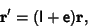(1)

where the Matrixis infinitesimal and I is the Identity Matrix. (Note that the infinitesimal transformation may not correspond to an inversion, since inversion is a discontinuous process.) The Commutativity of infinitesimal transformationsandis established by the equivalence of(2)(3)

Now let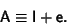(4)

The inverse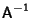is then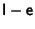, since(5)

Since we are defining our infinitesimal transformation to be a rotation, Orthogonality of Rotation Matrices requires that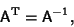(6)

but(7)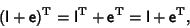(8)

soand the infinitesimal rotation is Antisymmetric. It must therefore have a Matrix of the form(9)

The differential change in a vectorupon application of the Rotation Matrix is then(10)

Writing in Matrix form,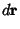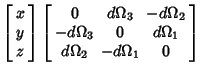(11)(12)

Therefore,(13)

where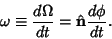(14)

The total rotation observed in the stationary frame will be a sum of the rotational velocity and the velocity in the rotating frame. However, note that an observer in the stationary frame will see a velocity opposite in direction to that of the observer in the frame of the rotating body, so(15)

This can be written as an operator equation, known as the Rotation Operator, defined as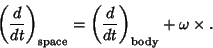(16)